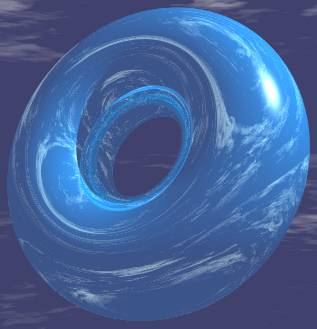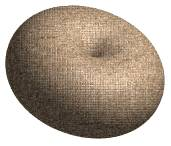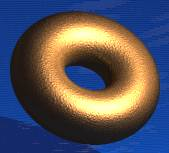# 环面• 可以用一个小圆（半径 r
沿着一个大圆（半径 R
移动来形成。
• 没有边或顶点
• 不是多面体## 表面积### 例子：r = 3，R = 7

 表面积 = 4 × π2 × R × r = 4 × π2 × 7 × 3 = 4 × π2 × 21 = 84 × π2 ≈ 829

## 体积

### 例子：r = 3，R = 7

 体积 = 2 × π2 × R × r2 = 2 × π2 × 7 × 32 = 2 × π2 × 7 × 9 = 126 π2 ≈ 1244（这个不是真正的罗马垫，这个只是我画出来的图）

## 更多环面图像